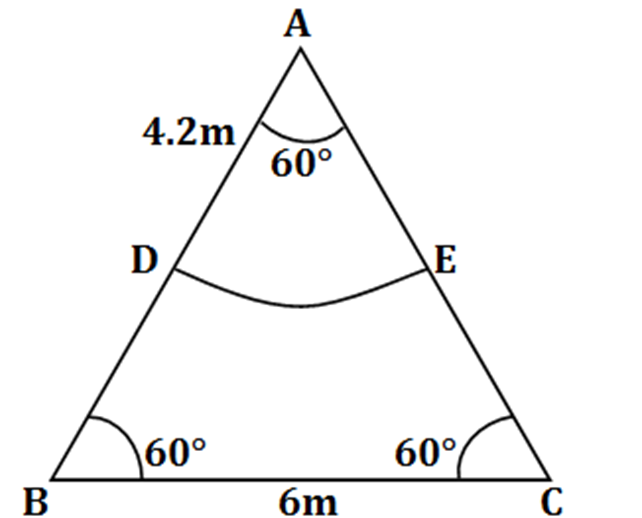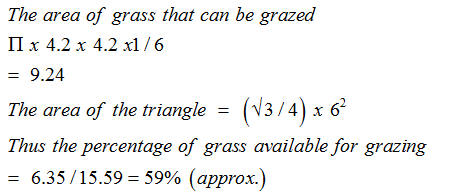• This is an assessment test.
• These tests focus on geometry and mensuration and are meant to indicate your preparation level for the subject.
• Kindly take the tests in this series with a pre-defined schedule.

## Geometry and Mensuration: Test 30

Congratulations - you have completed Geometry and Mensuration: Test 30. You scored %%SCORE%% out of %%TOTAL%%. You correct answer percentage: %%PERCENTAGE%% . Your performance has been rated as %%RATING%%
 Question 1
A grassy field has the shape of an equilateral triangle of side 6m. A horse is tied to one of its vertices with a rope of length 4.2m. The percentage of the total area of the field which is available for grazing is best approximated by
 A 50% B 55% C 59% D 62%
Question 1 Explanation:Question 2
The areas of two circles are in the ratio 1: 2. If the two circles are bent in the form of squares, what is the ratio of their areas?
 A 1: 2 B 1: 3 C 1: √2 D 1: 4
Question 2 Explanation:
Area of two circles in 1:2 then their perimeter will be in 1:√2 Since the circle is bent to form a square,
so the perimeter of square = circumference of the circle
So perimeters of squares will also be in 1:√2
So area of squares is 1²:(√2)²=1: 2
Correct option is (a)
 Question 3
A wall is of the form of a trapezium with height 4m and parallel sides being 3m and 5m. What is the cost of painting the wall, if the rate of painting is Rs.25 per sq m?
 A Rs.240 B Rs.400 C Rs.480 D Rs.800
Question 3 Explanation:
$\begin{array}{l}The\text{ }area\text{ }of\text{ }the\text{ }wall=\frac{1}{2}(3+5)4=16\,sq\,m\\The\text{ }total\text{ }cost\text{ }=\text{ }25\times 16=Rs.\text{ }400.\\Correct\text{ }option\text{ }is\text{ }\left( b \right)\end{array}$
 Question 4
The area of a rectangle whose length is 5 units more than twice its width is 75 sq units. What is the width?
 A 3 units B 5 units C 7 units D 10 units
Question 4 Explanation:
Let the width of the rectangle be x units and thus length = 2x+5.
Thus, (2x+5)x=75
=>x=5.
Correct option is (b).
 Question 5
The diameter of a circle circumscribing a square is 15√2cm. What is the length of the side of the square?
 A 15 cm B 12 cm C 10 cm D 7.5 cm
Question 5 Explanation:
The diameter of the circle = diagonal of the square. Let the side of the square is 'x' cm.
So x2 + x2 = (15√2)2 = 450
=> 2x2 = 450
=> x2 = 225
=> x = 15 cm
Thus the side= 15 cm Correct option is (a)
Once you are finished, click the button below. Any items you have not completed will be marked incorrect.
There are 5 questions to complete.
 ← List →
 1 2 3 4 5 End

### Want to explore more Arithmetic Tests?

Get Posts Like This Sent to your Email
Updates for Free Live sessions and offers are sent on mail. Don't worry: we do not send too many emails..:)
Get Posts Like This Sent to your Email
Updates for Free Live sessions and offers are sent on mail. Don't worry: we do not send too many emails..:)